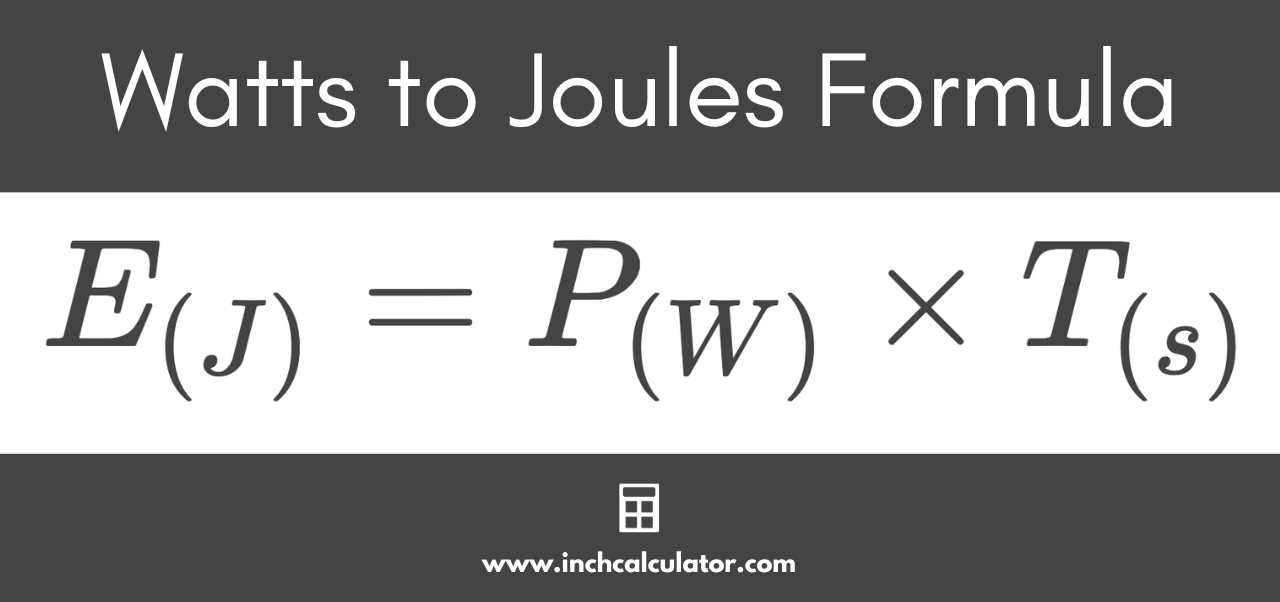# Watts to Joules Conversion Calculator

Convert watts to joules by entering the current and time in seconds using this easy calculator.

watts
seconds

## Energy:

joules
Learn how we calculated this below

Do you want to convert joules to watts instead?

## How to Convert Watts to Joules

Before we go over how to convert watts to joules, let’s cover some terminology that we’ll be using in the formula below.

Energy is the ability of a system to perform work, and it is measured in joules (J). One joule of energy is equal to the amount of energy dissipated to move a one-kilogram object one meter using a constant force of one newton.

Power is a measure of the rate at which work is performed in a system, and it’s measured in watts (W). One watt of power is equal to the rate at which one joule of energy is delivered in one second.

For example, a 100-watt light bulb will consume energy at a rate of 100 joules/second.

To convert watts to joules, you’ll need a formula.

### Watts to Joules Formula

The watts to joules formula is:

E(J) = P(W) × T(s)

Thus, the energy E in joules is equal to the power P in watts times the time T in seconds.

To calculate the joules of energy exerted, substitute the watts and time in seconds in the formula and solve.For example, let’s calculate the energy in joules for a 50-watt power consumption over 25 seconds.

E(J) = 50 W × 25 s
E(J) = 1,250 J

So, 50 watts of power drawn over 25 seconds is equal to 1,250 joules of energy.

You can also use our watts to amps converter to convert power to current.